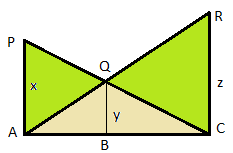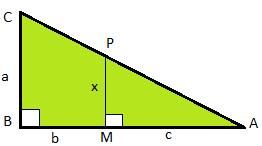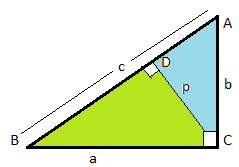### Common Errors in Secondary Mathematics

Common Errors Committed  by the  Students  in Secondary Mathematics   Errors  that students often make in doing secondary mathematics  during their practice and during the examinations  and their remedial measures are well explained here stp by step.  Some Common Errors in Mathematics

### Math Assignment Ch-6 Class X | Triangle

Maths. Assignment Class X Chapter 6 : Triangle
Extra questions of class x, chapter 6, extra questions of triangle chapter 6, class x
Important Result :

Internal bisector of angle of a triangle divides the opposite side internally in the ratio of the sides containing the angle.    In Triangle ABC, if AD is the bisector of ∠A then $\frac{AB}{AC}=\frac{BD}{DC}$

For detailed study and basic concepts related to the similarity of triangles: Click Here

Solve the following questions

 Question 1: Q. 1. PA, QB and RC are each perpendicular to AC thenQuestion 2:If the bisector of an angle of  triangle bisects the opposite side, then prove that the triangle is isosceles. Question 3:O is any point inside a triangle ABC. The bisectors of ∠AOB, ∠BOC and ∠COA meet the side AB, BC and CA in points D, E, F respectively. Show that AD X BE X CE = BD X EC X FA Question 4:In triangle ABC, AD is the internal bisector of ∠A which meets BC at point D. Prove that: Question 5:In the given figure express x in terms of a, b, cQuestion 6:Q6 The areas of two similar triangles are 81 cm2 and 49 cm2 respectively. Find the ratio of their corresponding heights? What is the ratio of their corresponding median. Question 7:In quadrilateral ABCD,  ∠B = 90o  If AD2 = AB2 + BC2 = CD2  Prove that ∠ACD = 90oQuestion 8:In the given figure PQR,   S and T trisects the side QR of a right triangle PQR. Prove that 8PT2 = 3PR2 + 5PS2Question 9:Triangle ABC is right angled at B ,  D is the mid- point of BC. Prove that AC2 =  4AD2 - 3AB2 Question 10:In triangle ABC, AD ⟂ BC and point D lies on BC such that 2BD = 3CD, Prove that 5AB2 = 5AC2 +BC2 Question 11:△ABC is right angled at C. Let BC = a, CA = b, BC = c, and let p be the length of perpendicular from C on ABProve That :Question 12:In △ABC, P and Q are the points on sides CA and CB respectively, which divides these sides in 2 : 1. Prove that (i) 9AQ2 = 9AC2 + 4BC2(ii) 9BP2 = 9BC2 + 4AC2(iii) 13AB2  = 9(AQ2 + BP2)Question 13:In △ABC, AC > AB,  D is the mid - point of BC and   AE⟂BC .Prove that :